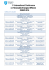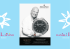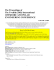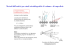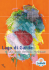# Why is the sky blue during day time, and red... Scattering of light !! 1

## Transcription

Why is the sky blue during day time, and red... Scattering of light !! 1
```Why is the sky blue during day time, and red at sunset or sunrise?
Scattering of light !!
1
Rayleigh Scattering
Scattering object << wave length Æ Harmonic Oscillator
Scattering cross section
8π d 6 ε − 1
σs =
3 λ4 ε + 2
2
σs
d: diameter of object
n: refractive index
λ : wavelength
450
600
λ
Scattering object : air molecules
earth
sun
air
2
When the size of the object is larger..
Mie scattering
Prism bulk
Prism,
Object size ~> wavelength
Object size >> wavelength
… cloud particle ~ several microns
… droplet
p of water
-White Cloud
scattering all the wavelength equally
- Brocken spectre (Brockengespenst)
wavelength dependent scattering by cloud
3
Pictures from Wikipedia
Contents
1. Dielectric constant of metals
(what is metal for light)
2. Surface Plasmon (bulk)
3. Localized surface plasmon resonance
Æ scattering
tt i off nano particle
ti l
4
Dielectric constant
ε=ε’+
’ i ε’’’’
Real:
Imaginary:
How much is polarized
polarized.
ÆReflection when negative
How much
H
h iis outt off phase
h
Æloss
D = εεE= ε0εrE
Transparent material
Lossy material
Metallic material
Reflectivity:
R=
ε r −1
εr +1
Æ ε: real, positive
Æ ε: complex
Æ ε: complex, negative real part
When εr <= 0, then R =1, 100% reflection
5
Drude model
Light (= electric field + magnetic field) interacts with electrons
d 2u m du
Equation of motion : m 2 +
= qE0 exp(−iωt )
dt
τ dt
Friction term :loss
Solution:
Polarization:
Induction:
u=−
m : effective mass of electron
u : position
t : time
q : elemental charge
g
E : field, E0exp(-iwt)
τ : relaxation time
q
1
E0 exp(−iωt )
m ω (ω + i / τ )
P= Nqu
P
Nq 2
1
P=−
E
m ω (ω + i / τ )
D = ε 0 E + P = ε 0ε r E
ωp2
ε r = 1−
ω (ω + i / τ )
Nq 2
ωp =
mε 0
Volume plasmon freq. (εr=0 without friction)
6
* Drude-Lorentz model: include “spring bouncing” term proportional to position u
5.0
Drude model
0.0
ε’ , ε’’
0.0
-5.0
2.0
4.0
6.0
eV
e_real
e_im
-10.0
-15.0
-20.0
Measured values
7
P.B.Johnson et al; Phys.Rev. B v6.n12 (1972), Phys.Rev. B v9.n12 (1974),
Surface Plasmon : Wave propagation on plane surface
z
Electric field is perpendicular to the surface
Dielectric(eg.water)
kp
x
Ey=0
metal
B.C.
C
kp = k
x
diel
=k
x
met
z
k diel
Tangential component ,
Speed of light in dielectric
Surface propagating wave:
k
x 2
diel
+k
z 2
diel
kp =
ω
c
⎛ω ⎞
= ε diel ⎜ ⎟
⎝c⎠
ε diel
=
z
k met
ε met
Normal component
2
ε met ε diel
ε met + ε diel
Dispersion relation
ε is also ω dependent
Resonance at
′ + ε diel = 0
ε met
ω sp = ω p
1
1 + ε diel
8
Dispersion relation of “free” wave in
dielectric medium (x component)
ω
ωill =
c
ε diel 2 ⋅ sin θ
⋅ kill − p
Dispersion relation of free wave in dielectric(θ<90°)
Dispersion relation of free wave in dielectric(θ=90°)
ωp
ωsp
Dispersion relation of SP
k
Mode should match to excite SP
Ædispersion
p
relation should intersect
Di l t i medium
Dielectric
di
εdiel
Metal thin film
prism
p
εprism > εdiel
Reflection intenssity
Kretschmann-configuration
angle
9
Localized Surface Plasmon
Structure size < Wavelength
There is no propagating wave at surface, but there is surface field…
Hard to describe the system with simple wave equations.
-Numerical simulation
-Analytical calculation on simplest system : sphere
Æ Mie Theory
10
Mie theory
Maxwell’s equation is linear
Total Field
=
Plane wave
(excitation)
+
Outgoing wave
+ Standing wave
(scattered wave)
(wave inside)
ÆSolve the coefficient of each wave, by matching boundary conditions
(same as wave guide theory)
Solve the wave equation on spherical coordinate
11
Mie theory
– spherical waves Maxwell eq.
Plane wave
∇× E = −
Wave eq:
(∇ 2 + k 2 )ψ (r ) = 0
ψ = Aeikr
∂B
∂t
∇⋅B = 0
∇× H =
∂D
∂t
∇⋅D = 0
Spherical wave
Wave eq in spherical coordinate:
⎡1 ∂ ⎛ 2 ∂ ⎞
r
1
∂ ⎛
∂ ⎞
1
∂2
2⎤
+
sin
i
θ
+
+
k
ψ
(
r
)=0
r
⎟ 2
⎜
⎟ 2
⎢ 2 ⎜
⎥
2
∂θ ⎠ r sin θ ∂φ
⎣ r ∂r ⎝ ∂r ⎠ r sin θ ∂θ ⎝
⎦
Solution 1: out going wave
ψ m ,n (kr ,θ , φ ) = hn (1) (kr ) Pnm (cos θ )eimφ
Infinite @ r=0
Solution 2: “regular”
regular wave (inside sphere)
Rgψ m ,n (kr , θ , φ ) = jn (kr ) Pnm (cos θ )e imφ
(1)
Finite @ r=0
General Solution : linear combination of all the special solutions
ψ = ∑∑ (K mn ⋅ψ mn + Lmn ⋅ Rgψ mn )
m
n
12
Simplified Scattering Model
for very small spherical particle
Assumption:
+
+
+
+
E
1st order in mulipole expansion
in Mie Theory
-
-
-
Dipole oscillator
Homogenous field
R l i h scattering
Rayleigh
tt i
8π d 6 ε − 1
σs =
3 λ4 ε + 2
2
Scattering Cross Section
3
σs =
2π
′ − ε diel ) 2 + (ε met
′′ ) 2
⎛ω4 ⎞ 2
2 (ε met
⎜⎜ ⎟⎟ε diel ⋅ V
2
2
′
′
′
c
(
ε
2
ε
)
(
ε
)
+
+
⎝ ⎠
met
diel
met
Extinction Cross Section
σ ext = 9
ω
c
(ε diel ) 3 ⋅ V
V : volume of particle
σ abs = σ ext − σ s
′′
ε met
′ + 2ε diel ) 2 + (ε met
′′ ) 2
(ε met
13
Comparison with normal SPR and LSPR
SPR
Resonance when
ε’met = -εdiel
kp =
LSPR (nanoparticle)
Resonance when
A
Au
ε’met = -2εdiel
σ ext = 9
ω
c
ω
c
ε met ε diel
ε met + ε diel
(ε diel ) 3 ⋅ V
′′
ε met
′ + 2ε diel ) 2 + (ε met
′′ ) 2
(ε met
10
8
εdiel = 2.25 (n=1.5)
6
4
2
0
200
er
300
400
500
600
ei 700
800
-2
-4
SPR ~520nm
-6
-8
LSPR ~550nm
-10
14
Calculated scattering cross section from simplified Mie theory
nglass or nwater
3
σs =
2π
20nm
Au
′ − ε diel ) 2 + (ε met
′′ ) 2
⎛ω4 ⎞ 2
2 (ε met
⎜⎜ ⎟⎟ε diel ⋅ V
2
2
′
′
′
c
(
ε
+
2
ε
)
+
(
ε
)
⎝ ⎠
met
diel
met
Scatttering cross s
section
n=1.5
n=1.33
400
450
500
550
600
650
700
750
800
Wavelength / nm
Resonance peak shifts by different surrounding medium
Æ sensing application
15
Simulated scattering spectrum and field map
E field map
10nm Au particle in water(n=1.33)
Sccattering in
ntensity
E
Field enhancement at resonance
450
500
550
600
650
Wave length
700
750
800
16
Literature
“Mie S
“Mi
Scattering”
tt i ”
R.M. Drake and J.E. Gordon
Am. J. Phys. 53 955 (1985).
“Scattering of Electromagnetic Waves –theories and applications”
Leung Tsang, Jin Au Kong, Kung-Hau Ding
2000 John Wiley&Sons, Inc.
“Nanophotonics with Surface Plasmons”
V.M. Shalaev, S.Kawata
2007 Elsevier
Etc…..
Useful software
“Mie Plot”
http://www philiplaven com/mieplot htm
http://www.philiplaven.com/mieplot.htm
17
Appendix
• Overview of Mie Theory
18
Mie theory
– spherical waves Maxwell eq.
Plane wave
∇× E = −
Wave eq:
(∇ 2 + k 2 )ψ (r ) = 0
ψ = Aeikr
∂B
∂t
∇⋅B = 0
∇× H =
∂D
∂t
∇⋅D = 0
Spherical wave
Wave eq in spherical coordinate:
⎡1 ∂ ⎛ 2 ∂ ⎞
r
1
∂ ⎛
∂ ⎞
1
∂2
2⎤
+
sin
i
θ
+
+
k
ψ
(
r
)=0
r
⎟ 2
⎜
⎟ 2
⎢ 2 ⎜
⎥
2
∂θ ⎠ r sin θ ∂φ
⎣ r ∂r ⎝ ∂r ⎠ r sin θ ∂θ ⎝
⎦
Solution 1: out going wave
ψ m ,n (kr ,θ , φ ) = hn (1) (kr ) Pnm (cos θ )eimφ
Infinite @ r=0
Solution 2: “regular”
regular wave (inside sphere)
Rgψ m ,n (kr , θ , φ ) = jn (kr ) Pnm (cos θ )e imφ
(1)
Finite @ r=0
General Solution : linear combination of all the special solutions
ψ = ∑∑ (K mn ⋅ψ mn + Lmn ⋅ Rgψ mn )
m
n
19
How do these functions look like?
Rgψ m ,n (kr , θ , φ ) = jn (kr ) Pnm (cos θ )e imφ
(1)
ψ m ,n (kr ,θ , φ ) = hn (kr ) Pnm (cos θ )eimφ
(1)
Legendre function
n+ m
(−1) m
2 m/2 d
2
n
P ( x) = n (1 − x )
(
x
−
1
)
2 n!
dx n + m
m
n
Spherical Harmonics
Ynm = N ⋅ Pnm (cos θ )e imφ
n
Same as electron orbits around atom model !
m
Hankel function
hn ( x)= jn ( x) + iyn ( x)
(1)
jn (x) :
yn (x) :
Bessel function of 1st kind
Bessel function of 2nd kind
20
Bessel function
Relation like exp(ix) and sin(x), exp(x) and sinh(x)
Hankel function
21
Bessel funciton
1 t kind
1st
ki d
2nd kind
infinite
22
Scattering of sphere : sphere + plane wave
….very different symmetries
Plane wave on spherical coordinate, or spherical wave on cartesian coordinate?
How to express plane wave in spherical coordinate?
Vector spherical harmonics: ~ 3 base vector in harmonics
23
Define 3 vector spherical wave functions:
With Rg : Bessel function based
Without Rf : Hankel function based
Expression of Plane wave traveling on z axis :
24
Now, finally scattering!
Wave inside
Possible waves in the scattering event
Subscript p
Plane wave
Scattered wave
Incident plane wave field
scattered wave field
Internal field
Outside the sphere
Outside the sphere
inside the sphere
25
Solve at the boundary
BC
B.C.
Continuity of
a
and
(or
)
at r = a
Compare the coeffcient of M, N Æ solve a,b,c,d
k : outside
k : inside
kp
i id sphere
h
26
z
Set the field along the axis for simplicity
Scattering field:
S1
illumination
θ
y
x
E
E
S2
φ
Unpolarized(sum of the two above) :
Scattering cross section (Integrate (Es)2 around the sphere)
Scattering efficiency
27
Extinction…..
Scattering object : Perfect Electric Conductor
=
+
Incoming plane wave
Total field
Scattered field
Small field due to interference
Extinction Æ out of phase component in forward scattering Æ imaginary part
Forward scattering ( θ = 0 )
Extinction cross section
Destructive component : Imaginary part of forward scattering x incident
28
How to measure ???
Lambert-Beer ‘s law
Definition of “extinction” = “scattering” + ”absorption”
Macroscopic
z
I0
I
α: extinction (absorption) coeff.
Microscopic view
Detect only scattered wave : scattering spectrum
Scattering object
Detect only forward wave
: extinction spectrum
Incoming wave
29
Rayleigh Scattering
⎛ d 2u
2 ⎞
m⎜⎜ 2 + ω0 u ⎟⎟ = qE0 exp(−iωt )
⎠
⎝ dt
Harmonic Oscillator
u=−
Polarization :
Fi ld ffrom di
Field
dipole
l :
(r>>u)
Poynting vector :
qE
qE
E0
E0
exp(
−
i
ω
t
)
→
−
exp(−iωt )
2
2
2
m(ω0 − ω )
mω 0
ω<<ω0
q 2 E0
p = q ⋅u = −
2
mω0
ω2
[n × (n × p )]exp((ik ⋅ r − iωt )
Es (r , t ) = −
2
4πε 0 c r
Ss =
Es × Bs
μ0
=
Es
k
k
2
cμ 0
n
Illumination :
2
4
ω 4 q E0
2
<
S
>
>=
sin
Θ
s
4
2
2
3 2
Time average:
32π ε 0 c r ω0
m
1
n=
< Si >=
>
E0
2
2cμ 0
30
ks
Θ
dipole
n = (sin θ ,0, cos θ )
coordinate
E 0 = E0 (cosψ , sinψ ,0)
x
ki
cos Θ =
z
n ⋅ E0
= cosψ sin θ
E0
2
Segment of cross section:
σ =∫
2π
0
dψ ∫
π
0
4
⎞ ω4
dσ < S s > ⎛
ω
q2
2
2
⎟
=
= ⎜⎜
sin
Θ
=
a
sin
Θ
0
4
4
2
2 ⎟
dΩ < Si > ⎝ 4πε 0 mc ⎠ ω0
ω0
8π ω 4 2
sin θ dθ ⋅ a0 sin Θ =
a
4 0
3 ω0
2
For spherical object
p = 4πε 0
a
(ε − ε 0 )a
E0
2ε 0 + ε
3
q 2 E0
p = q ⋅u = −
2
mω0
Lorentz-Lorenz model
σ=
8π ω 6 ⎛ ε − ε 0
a ⎜⎜
4
3 c
⎝ 2ε 0 + ε
4
⎞
⎟⎟
⎠
2
31
```

### DAFTAR PUSTAKA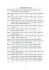### Mapa Esquemático### • • ! • r " "~ r," os rCyernID.en\ usctC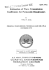### Lecuture 3 Estimating surface rain rate from satellite passive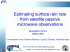### 2gh usm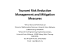### RENEW - schedule at a glance